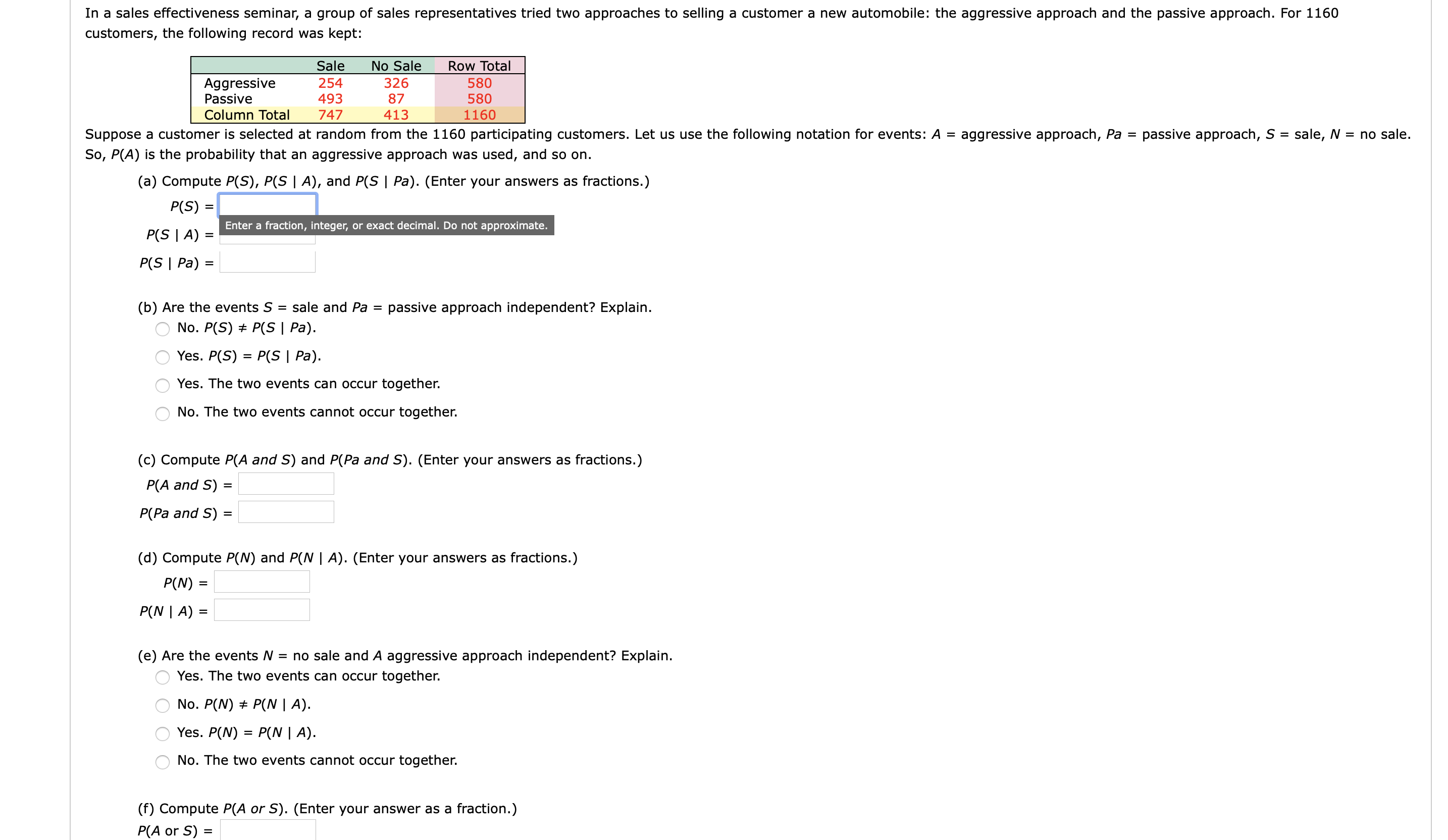# In a sales effectiveness seminar, a group of sales representatives tried two approaches to selling a customer a new automobile: the aggressive approach and the passive approach. For 1160customers, the following record was kept:SaleNo SaleRow Total254326580AggressivePassive87580493Column Total4137471160passive approach, S = sale, N = no sale.Suppose a customer is selected at random from the 1160 participating customers. Let us use the following notation for events: A =aggressive approach, Pa =So, P(A) is the probability that an aggressive approach was used, and so on.(a) Compute P(S), P(S | A), and P(S | Pa). (Enter your answers as fractions.)P(S) =Enter a fraction, integer, or exact decimal. Do not approximate.P(S | A) =P(S | Pa) =sale and Pa =(b) Are the events Spassive approach independent? Explain.No. P(S) + P(S | Pa).Yes. P(S) = P(S | Pa).Yes. The two events can occur together.No. The two events cannot occur together.(c) Compute P(A and S) and P(Pa and S). (Enter your answers as fractions.)P(A and S) =%3DP(Pa and S) =(d) Compute P(N) and P(N | A). (Enter your answers as fractions.)P(N)P(N | A) =(e) Are the events N = no sale and A aggressive approach independent? Explain.Yes. The two events can occur together.No. P(N) + P(N | A).Yes. P(N) = P(N | A).No. The two events cannot occur together.(f) Compute P(A or S). (Enter your answer as a fraction.)P(A or S) =%3D

Question
43 viewshelp_outlineImage TranscriptioncloseIn a sales effectiveness seminar, a group of sales representatives tried two approaches to selling a customer a new automobile: the aggressive approach and the passive approach. For 1160 customers, the following record was kept: Sale No Sale Row Total 254 326 580 Aggressive Passive 87 580 493 Column Total 413 747 1160 passive approach, S = sale, N = no sale. Suppose a customer is selected at random from the 1160 participating customers. Let us use the following notation for events: A = aggressive approach, Pa = So, P(A) is the probability that an aggressive approach was used, and so on. (a) Compute P(S), P(S | A), and P(S | Pa). (Enter your answers as fractions.) P(S) = Enter a fraction, integer, or exact decimal. Do not approximate. P(S | A) = P(S | Pa) = sale and Pa = (b) Are the events S passive approach independent? Explain. No. P(S) + P(S | Pa). Yes. P(S) = P(S | Pa). Yes. The two events can occur together. No. The two events cannot occur together. (c) Compute P(A and S) and P(Pa and S). (Enter your answers as fractions.) P(A and S) = %3D P(Pa and S) = (d) Compute P(N) and P(N | A). (Enter your answers as fractions.) P(N) P(N | A) = (e) Are the events N = no sale and A aggressive approach independent? Explain. Yes. The two events can occur together. No. P(N) + P(N | A). Yes. P(N) = P(N | A). No. The two events cannot occur together. (f) Compute P(A or S). (Enter your answer as a fraction.) P(A or S) = %3D fullscreen
check_circle

Step 1

Since you have posted a question with multiple sub-parts, we will solve first three sub-parts for you. To get remaining sub-part solved please repost the complete question and mention the sub-parts to be solved.

Step 2
Step 3

a)

The probability of sale can be calculated as,

The required probabilities...

### Want to see the full answer?

See Solution

#### Want to see this answer and more?

Solutions are written by subject experts who are available 24/7. Questions are typically answered within 1 hour.*

See Solution
*Response times may vary by subject and question.
Tagged in

### Statistics